#Physics Problems with Solutions# Free SAT II Physics Detailed Solutions Sample 1

 Solutions to the sat physics problems with detailed explanations. A particle moves 80 cm South in 0.12 seconds and then 60 cm West in 0.08 seconds. What is the magnitude of the average velocity of the particle? A) 700 cm/s B) 1417 cm/s C) 500 cm/s D) 250 cm/s E) 709 cm/s Solution To find the average velocity, we need the displacement and time. The path of the particle follows the two sides of a right triangle and therefore the magnitude of the displacement $d$ is the hypotenuse of the right triangle with legs $80$ and $60$. Use Pythagora's theorem $\text{d}^2=80^2+60^2$ which gives d = $100$ cm $\text{velocity} = \dfrac{\text{displacement}}{\text{time}} = \dfrac{100 cm}{0.12 s + 0.08 s} = 500$ cm/s When a car's speed changes from 20 m/s to 40 m/s, its kinetic energy A) stays the same B) is doubled C) is halved D) is tripled E) is quadrupled Solution The kinetic energy E of a particle having constant speed v and mass m is given by $E=\dfrac{1}{2}mv^2$ In the above, the speed changes from 20 to 40 m/s, hence doubles If we double v in the formula for E above, we get $E' = \dfrac{1}{2}m(2v)^2 = 2mv^2$ The energy is multiplied by 4, hence is quadrupled. The graph below is that of the velocity of a moving particle. At which of the points A, B, C, D or E is the acceleration highest?A) A B) B C) C D) D E) E Solution The acceleration is the change of velocity over time and therefore a larger change of the velocity v means a larger acceleration. I we examine the given graph, the velocity v changes rapidly at point A (tangent at that point is the steepest) and therefore the acceleration is highest at point A. The graph below is that of the height of a ball thrown vertically upward. At which point is the velocity close or equal to zero?A) A B) B C) C D) D E) E Solution The velocity is the change of height with the time. At point C, there is no change of h (tangent at that point is horizontal and its slope is equal to zero). Therefore the velocity is close to or equal to zero at point C. The capacitors between A and B and C and D are equivalent and have capacitance C each. How many equivalent capacitors, with capacitance C, we need to put in series between E and F so that the total capacitance in the circuit is equal to 2.5C?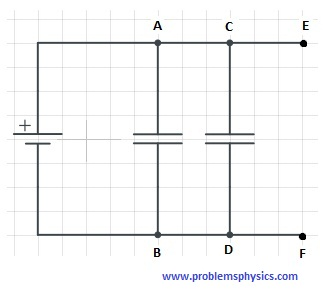A) 2 B) 3 C) 4 D) 5 E) 6 Solution Let Ceq be the capacitance of the capacitors between point E and F. Then we have 3 capacitors in parallel whose equivalent capacitance is given by C + C + Ceq and is equal to 2.5C. Therefore Ceq = 0.5C If we now put n capacitors of capacitance C in series between E and F, we have 1 / Ceq = 1/C + 1/C + 1/C + ... + 1/C = n/C Ceq = C/n Ceq = 0.5C = C/n n = 2 All resistors in the circuit below are equivalent. What is the ratio of the voltage drop between points A and B and the voltage drop between points B and C?A) 1/3 B) 1/9 C) 1/6 D) 1/2 E) 1 Solution All resistors are equivalent and therefore have the same resistance R. Three resistors in series are equivalent to 3R. Let Req be the resistor equivalent to the groups of resistors 3R between B and C, then we have 1 / Req = 1/3R + 1/3R + 1/3R = 3/3R = 1/R Hence Req = R So the resistors between A and B and B and C are equivalent and therefore the voltage drop between A and B and B and C are equal and therefore their ratio is equal to 1 Vectors u and v have magnitudes of 15 and 7 units respectively. What is the possible highest value of the magnitude of the vector 3u - 2v? A) 31 units B) 45 units C) 66 units D) 59 units E) 8 units Solution The magnitude of vector 3u is equal to 3*15 = 45 and the magnitude of -2v is equal to 2*14 = 14 The magnitude is maximum when vectors 3u and -2v are parallel and opposite in which case the magnitude of 3u - 2v = 45 + 14 = 59 In order to increase the frequency of oscillations, for small amplitudes, of a pendulum of length L with a bob of mass M, you need to A) increase L B) decrease L C) increase M D) decrease M E) decrease both M and L Solution The period T of a pendulum of length L is given by $T=2\pi\sqrt{\dfrac{L}{g}}$ If F is the frequency, then $F = 1/T = \dfrac{1}{2\pi}\sqrt{\dfrac{g}{L}}$ In order to increase F we have to decrease L. What is the tension in the upper string of the system made up of two blocks of metal each of mass 5 kg, suspended from the ceiling by strings of negligible mass, as shown above.A) 49 N B) 25 N C) 980 N D) 98 N E) 10 N Solution The tension $T$ in the upper string is the force exerted by the string to have equilibrium. Hence the magnitude of $T$ is equal to the weights of both masses $T = 5\cdot g + 5\cdot g = 98$ N In the figure below, is shown mass M attached to a spring. The initial position of mass M is at point C. A and B are the extreme positions of the mass as it oscillates. The kinetic energy of mass M is maximum at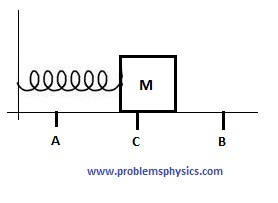A) point A only B) point B only C) point C only D) points A and B E) points C and B Solution At points A and B the mass stops to change direction and therefore the velocity at each of these two points is equal to zero and therefore the kinetic energy at each of these points is equal to zero (the potential energy is maximum). At point C, all of the potential energy has been converted into kinetic energy and therefore the kinetic energy is maximum at point C. What is the y-component of the resultant force when 3 forces, whose magnitudes are given, are acting on an object at point O as shown in the figure?A) 12 cos(α) + 20 sin(θ) B) 12 sin(α) + 20 sin(θ) C) -10 D) 12 sin(α) + 20 sin(θ) - 10 E) 12 cos(α) + 20 sin(θ) - 10 Solution Let us calculate the x component $x_c$ and y component $y_c$ component of each force Force of 20 N: $x_c = 20 cos(\theta)$ ; $y_c = 20 sin(\theta)$ Force of 12 N: $x_c = -12 sin(\alpha)$ ; $y_c = 12 cos(\alpha)$ Force of 10 N: $x_c = 0$ ; $y_c = -10$ The y component of the resultant force is the sum of all 3 y components of the acting forces and is given by $20 sin(\theta) + 12cos(\alpha) - 10$ The graph of the velocity against the time of a moving object is shown below. What is the displacement between t = 3 and t = 5?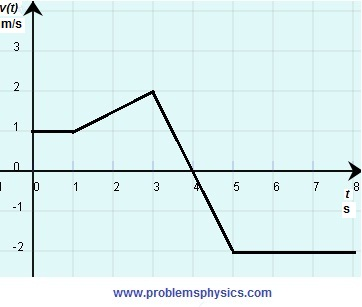A) 4 m B) 2 m C) equal or close to zero D) -4 m E) -2 m Solution The displacement between t = 3 and t = 5 is the total area of the surface between the curve of v and the x axis taking into account that the area of a surface below the axis is negative and the area of a surface above the x axis is positive. Hence the area of the triangle from t = 3 to t = 4 is equal to (1/2)*2*1 = 1 and the area of the triangle from t = 4 to t=5 is equal to -(1/2)*2*1 = -1 and the sum of the two areas is equal to zero. Three particles with charges +q at points A, -q at point B and +q at point C, are located at vertices of an equilateral triangle and the line through A and B is parallel to the x axis. What is true about the resultant force acting on the charge at point C?A) its x and y components are equal B) its y component is equal to zero C) its x component is equal to zero D) its x and y components are equal to zero E) its direction makes an angle of 45 degrees with the x axis Solution The two forces acting on the charge at point C are shown below. Because of the sides of an equilateral being equal, and the charges are equal in magnitude, the magnitude of all forces acting on C are equal. Since AB is parallel to the x axis, the angles made by the two forces acting at C with the x axis are equal in magnitude and of opposite signs and therefore the vertical component of the resultant force is equal to zero. All the other statements about the resultant are incorrect. Answer B.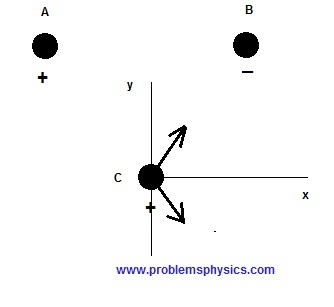What is the momentum of a moving object that has a mass of 100 Kg and kinetic energy of 20,000 Joules? A) 10,000 Kg.m/s B) 100 Kg.m/s C) 1000 Kg.m/s D) 2000,000 Kg.m/s E) 2000 Kg.m/s Solution Kinetic energy is given by $\dfrac{1}{2}mv^2 = 20,000$ and $m=100$ could be used to find $v^2=400$ and $v=20$ m/s Hence the momentum $p = mv = 100 Kg * 20$ m/s $= 2,000$ Kg.m/s Ball $A$ of mass $m$ and velocity $v$ collides with ball $B$ of mass m and velocity $-v$. What are the velocities $V_A$ and $V_B$ of the two balls after collision? A) $V_A=-v$ and $V_B=v$ B) $V_A=v$ and $V_B=-v$ C) $V_A=-v$ and $V_B=-v$ D) $V_A=v$ and $V_B=v$ E) $V_A=0$ and $V_B=v$ Solution The momentum of the two balls before collision is given by $mv + m(-v) = 0$. Let $V_A$ and $V_B$ are the velocities of A and B after collision, since the momentum is conserved then we can write $mv+m(-v)=mV_A + mV_B$ which simplifies to $mV_A + mV_B=0$, which gives $V_A = -V_B$ Conservation of kinetic energy gives: $\dfrac{1}{2}mv^2+\dfrac{1}{2}mv^2=\dfrac{1}{2}mV_A^2+\dfrac{1}{2}mV_B^2$ Substitute $V_B$ by $-V_A$ above and simplify to $v^2 = V_A^2$ which give $V_A=\pm v$ Since ball A will go in opposite direction after collision, $V_A=-v$ and $V_B=v$ The distance between two successive crests of a wave is $10^{-12}$ meters. The time between the arrival of successive crests is equal to $10^{-11}$ seconds. What is the speed of the wave? A) 100 m B) 0.1 mm C) 10 cm D) 10 mm E) 1 km Solution The distance between two crests is equal to the wavelength of the wave and the period of time between two successive crests is the period of the wave. The speed v is given by $v =\dfrac {wavelength}{period}= \dfrac{10^{-12}m}{10^{-11}s} = 0.1 m/s = 10 cm/s$ What is the gravitational force, to the nearest Newton, on a satellite of mass 100 kilograms in an orbit h of 38,400 kilometers?(The radius R of the earth is approximately equal to 6,400 kilometers) A) 27 N B) 980 N C) 1000 N D) 163 N E) 6000 N Solution Let $m_1$ be the mass of the satellite. The gravitational force $F_1$ of the satellite on the earth is given by $F_1=\dfrac{Gm_1m_2}{R^2}=m_1*g=100*9.8=1=980 N$ The gravitational force $F_2$ of the satellite at h = 38,400 km is given by $F_2=\dfrac{Gm_1m_2}{h^2}$ The ratio of the two forces $\dfrac{F_2}{F_1} = \dfrac{\dfrac{Gm_1m_2}{h^2}}{\dfrac{Gm_1m_2}{R^2}} = \dfrac{R^2}{h^2}=\dfrac{38,400}{6,400}=\dfrac{1}{36}$ $F_2=\dfrac{980}{36}=27 N$ (rounded) What will happen to the pressure of a gas in a closed container if its temperature (in degree Kevin) is doubled? A) it is halved B) it is doubled C) it stays the same D) it decreases E) it is quadrupled Solution For a fixed volume, the pressure is proportional to the temperature. If the temperature is doubled, the pressure is doubled. A ray of light is incident at an angle of $21.8^o$ on the interface between a material of refractive index $n_1$ and a material of refractive index $n_2$ as shown in the figure below. What is the measure of angle $x$ that the emerging ray makes with the perpendicular to the second interface?(Assume the interfaces are parallel)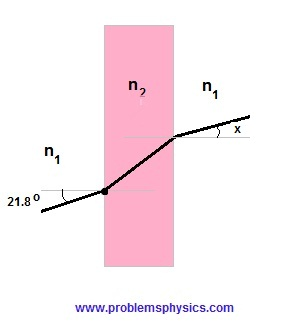A) $\dfrac{n_1}{n_2}21.8^o$ B) $\dfrac{n_2}{n_1}21.8^o$ C) $43.6^o$ D) $45^o$ E) $21.8^o$ Solution Apply Snell's law at each interface. $n_1 sin(21.8^o)=n_2 \sin(A)$ (interface on the left) $n_2 sin(B) = n_1 \sin(x)$ (interface on the right) A is the angle of refraction at the (on the left) interface $n_1 - n_2$ and B is the angle of incidence at the (on the right) interface $n_2 - n_1$. Angles A and B are equal since the interfaces are parallel. which gives $n_1\sin(21.8) = n_1\sin(x)$ and $x=21.8^o$ What is the energy of a photon with frequency $2.0 \times 10^{17}$Hz?(Plank constant $h = 6.6 \times 10^{-34}$J.s) A) $3.3 \times 10^{-51}$ J B) $6.6 \times 10^{-51}$ J C) $1.32 \times 10^{-17}$ J D) $1.32 \times 10^{-16}$ J E) $8.6 \times 10^{-17}$ J Solution The energy $e$ of a photon of frequency f is given by $e=hf=2.0 \times 10^{17} \cdot 6.6 \times 10^{-34}=1.32 \times 10^{-16}$ J Answers to the Above Questions C E A C A E D B D C E C B E A C A B E D